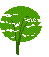Cross-referencing

From: Matthias Clasen <mclasen@sun2.mathematik.uni-freiburg.de>
Date: Thu, 27 Mar 1997 23:09:09 +0100 (MET)
```Hello all,
I have a simple question: Is it possible to process cross-references with
just the Core Query Language currently present in Jade ?

The situation is the following: My document looks like

<theorem id=foo> ... </theorem>

<proof of=foo> ... </proof>

(`id' is an ID attribute, `of' an IDREF)

I could something like

(element THEOREM
(make paragraph
(literal (append-string "Theorem "
(number->string (child-number))
"."))
(process-children)))

to give my theorem a running number. But how do I generate the
corresponding number for the proof ? My study of the standardbrought up
the following solution:

(element PROOF
(make paragraph
(literal "Proof of "
(number->string (child-number (referent)))
":"))
(process-children)))

but Jade chokes on `referent', which is not part of the Core Query
Language. Any ideas?